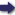(London :  Kegan Paul, Trench, Trübner & Co.,  1910.)

 Tools

## Search this bookPrev Page 53 Next``` CHAPTER LIII. 53 Add 38 to the lower number and multiply the sum by II. Divide the product by 703, and subtract the quo¬ tient from the upper number. The remainder in the upper place is the number of the civil days, and the remainder in the lower place is the number of the avctmas. Add i to the number of days and divide the sum by 7. The remainder shows the day of the week on which the date in question falls." This method would be correct if the months of the seventy-two years with which the calculation begins were lunar. However, they are solar months, in which nearly twenty-seven months must be intercalated, so that these seventy-two years are more than 864 months. We shall again exemplify this method in the case of Application our gauge-date, i.e. the beginning of Eabi' I., A.ii. 422. method to Between the above-mentioned ist of Sha'ban and the date^^"^^ latter date there have elapsed 2695 months. Adding these to the number of months adopted by the author of the method (864), you get the sum of 3559 months. Write down this number in two places. Multiply the one by 7, and divide the product by 228. The quotient represents the ctdhimdsa months, viz. 109. Rage 229. Add them to the number in the other place, and you get the sum 3668. Multiply it by 30, and you get the product 110,040. Write down this number in two different places. Add to the lower number 38, and you get 110,078. Multiply it by 11 and divide the product by 703. The quotient is 1722 and a remain¬ der of 292, i.e. the ctvctmas. Subtract the quotient from the upper number, and the remainder, 108,318, repre¬ sents the civil days. This method is to be amended in the following way : Emendation You must know that between the epoch of the era here method. used and the first of Sha'ban, here adopted as a date, there have elapsed 25,958 days, i.e. 876 Arabic months, or seventy-three years and two months. If we further ```Prev Page 53 Next